# A small circular loop of area 2 cm2 is placed in the plane of, and concentric with, a large circular loop of radius 1 m. The current in the large loop is changed at constant rate from 200A to -200A ( a change in direction) in a time of 1 s, starting at t=0. What is magnitude of the magnetic field at the center of the small loop due to the current in the large loop at (a) t=0, (b) t=0.5 s, and (c) t=1 s? (d) from t=0 to t=1 s, is reversed? Because the inner loop is small, assume is uniform over its area. (e) What emf is induced in the small loop at t=0.5 s?

9 years ago

Dear Sandeep,

a)
The equation for the current as a function of time is given by
I = I0 (1 - 2t)
The magnetic field at the center of a current loop is given by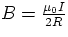At t = 0, the current is 200 A, so the magnetic field is given by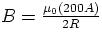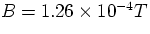At t = .5, the current is 0 A, so the magnetic field is zero. At t = 1, the current is -200 A, so the magnetic field is given by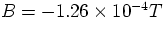b)
The magnetic flux through the loop is given by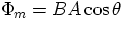Here, we take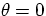.
So, the flux is given by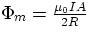The area of the loop is given by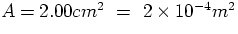So, the change in magnetic flux over time is given by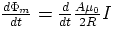Since A, R, and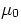are constants in time, this becomes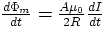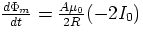Well, the change in magnetic flux over time is known as the EMF, so we write it as such.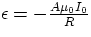Plugging in the numbers, we find that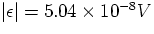Cracking IIT just got more exciting,It s not just all about getting assistance from IITians, alongside Target Achievement and Rewards play an important role. ASKIITIANS has it all for you, wherein you get assistance only from IITians for your preparation and win by answering queries in the discussion forums.

So start the brain storming…. become a leader with Elite Expert League ASKIITIANS

Thanks

Aman Bansal